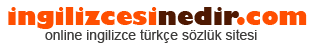ingilizce türkçe sözlük sitesine hoşgeldiniz.| Ana Sayfaİngilizce Türkçe Sözlükte Ara: Türkçe->İngilizce İngilizce->Türkçe * Aramak istediğiniz kelimeyi yazıp dil seçimini seçtikten sonra kelimenin anlamını öğrenebilirsiniz.

Kelime Anlamı

## Türkçeden İngilizceye Çeviri Sonucu

### volt Anlamı

• volt.
• A unit that measures the amount of electrical pressure. a unit of potential equal to the potential difference between two points on a conductor carrying a current of 1 ampere when the power dissipated between the two points is 1 watt; equivalent to the potential difference across a resistance of 1 ohm when 1 ampere of current flows through it.
• The unit of measure for voltage Voltage is the electrical pressure which forces the current to flow in a conductor such as a wire Volt-Ampere Voltage multiplied by the current ; apparent power For instance, a device rated at 10 amps and 120 V has a VA rating of 1200 or 1 2 kVA. the unit of electrical potential defined as one joule of work per coulomb of charge transferred.
• Unit of electromotive force Amount of force required to drive a steady current of one ampere through a resistance of one ohm current of one a. the unit of electrical potential defined as one joule of work per coulomb of charge transferred.
• A unit of electromotive force.
• The unit of voltage or potential difference. a unit of measurement for electrical pressure; named after the Italian scientist Alessandro Volta. the unit of electrical potential.
• The unit of measurement of electromotive force necessary to produce one ampere of current in a circuit having a total resistance of one ohm The volt is named for Alessandro Volta, an 18th century Italian physicist. measure of electrical potential, 110-volt house electricity has more potential to do work than an equal flow of 12-volt electricity.
• The unit of electrical potential.
• The unit of potential difference; joules per coulomb Electrical 'pressure '.
• A unit of electrical pressure It measures the force or push of electricity Volts represent pressure, correspondent to the pressure of water in a pipe A volt is the unit of electromotive force or electric pressure analogous to water pressure in pounds per square inch It is the electromotive force which, if steadily applied to a circuit having a resistance of one ohm, will produce a current one ampere.
• Unit of potential difference or electromotive force One volt is the potential difference needed to produce one ampere of current through a resistance of one ohm Voltage is the driving force throughout a sound system from the microphone input to the speaker terminals.
• The quantitative unit of measurement of electrical voltage.
• The standard unit of electromotive force or electrical pressure One volt is the amount of pressure that will cause one ampere of current to flow through one ohm of resistance.
• A VOLT is a unit of electrical pressure measuring the force or push of electricity.
• A unit of measure for electrical potential.
• The meter-kilogram-second unit of electromotive force, or potential difference; the potential difference between two points in an electric field such that one JOULE of work moves a charge of one COULOMB between these points The electrical force that, if steadily applied to a circuit with a resistance of one OHM, will produce a current of one ampere.
• The derived SI unit of electric potential difference.
• A unit of electrical pressure One volt is the amount of pressure that will cause one ampere of current in one ohm of resistance.
• The unit of electrical potential One volt is the electrical potential that will cause one ampere of current to flow through one ohm of resistance.
• volt.
• A sudden movement to avoid a thrust.
• A circular tread; a gait by which a horse going sideways round a center makes two concentric tracks.
Rastgele Türkçe Kelimeler